# 2.2.2 Polygons II, PT3 Practice

Question 6:
In diagram below, PQRSTU is a regular hexagon QUV is a straight line.Find the value of x.

Solution:

Question 7:
In diagram below, PQRSTU is a hexagon. UPE, PQF, QRG, RSH and UTJ are straight lines.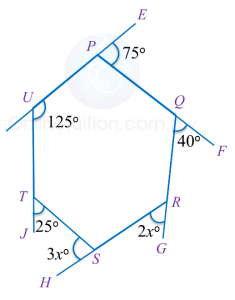Find the value of x.

Solution:

Question 8:
In Diagram below, A, B, C, D and E are vertices of a 9 sided regular polygon.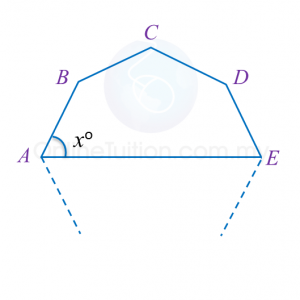Find the value of x.

Solution:

# 2.2.1 Polygons II, PT3 Practice

2.2.1 Polygons II, PT3 Practice

Question 1:
Diagram below shows a pentagon PQRST. TPU and RSV are straight lines.
Find the value of x.

Solution:

Question 2:
In Diagram below, PQRSTU is a hexagon. APQ and BTS are straight lines.

Solution:

$\begin{array}{l}\angle QPU={180}^{o}-{160}^{o}={20}^{o}\\ \text{Reflex}\angle PUT={360}^{o}-{80}^{o}={280}^{o}\\ \angle UTS={180}^{o}-{120}^{o}={60}^{o}\\ \angle TSR={180}^{o}-{35}^{o}={145}^{o}\\ \\ \text{Sum of interior angles of a hexagon}\\ =\left(6-2\right)×{180}^{o}\\ ={720}^{o}\\ \\ {x}^{o}+{y}^{o}+{145}^{o}+{60}^{o}+{280}^{o}+{20}^{o}={720}^{o}\\ {x}^{o}+{y}^{o}={720}^{o}-{505}^{o}\\ \text{}={215}^{o}\\ \text{}x+y=215\end{array}$

Question 3:
Diagram below shows a regular hexagon PQRSTU. PUV is a straight line.
Find the value of x + y.

Solution:
$\begin{array}{l}\text{Size of each interior angle of a regular hexagon}\\ =\frac{\left(6-2\right)×{180}^{o}}{6}\\ ={120}^{o}\\ {x}^{o}=\frac{{180}^{o}-{120}^{o}}{2}={30}^{o}\\ {y}^{o}={180}^{o}-{120}^{o}\\ \text{}={60}^{o}\\ {x}^{o}+{y}^{o}={30}^{o}+{60}^{o}\\ \text{}={90}^{o}\\ \text{}x+y=90\end{array}$

Question 4:
In the diagram below, KLMNP is a regular pentagon. LKS and MNQ are straight lines.

Solution:
$\begin{array}{l}\text{Size of each interior angle of a regular pentagon}\\ =\frac{\left(5-2\right)×{180}^{o}}{5}\\ ={108}^{o}\\ \angle PKS=\angle PNQ={180}^{o}-{108}^{o}={72}^{o}\\ \text{Reflex angle}\angle KPN={360}^{o}-{108}^{o}={252}^{o}\end{array}$

$\begin{array}{l}\text{Sum of interior angles of a hexagon}\\ =\left(6-2\right)×{180}^{o}\\ ={720}^{o}\\ \therefore {x}^{o}+{y}^{o}+{72}^{o}+{252}^{o}+{72}^{o}+{100}^{o}={720}^{o}\\ {x}^{o}+{y}^{o}={720}^{o}-{496}^{o}\\ \text{}={224}^{o}\\ \text{}x+y=224\end{array}$

Question 5:
In Diagram below, PQR is an isosceles triangle and PRU is a straight line.
Find the value of x + y.

Solution:
$\begin{array}{l}{x}^{o}={180}^{o}-{20}^{o}-{20}^{o}={140}^{o}\\ \angle PRS={180}^{o}-{110}^{o}={70}^{o}\\ {y}^{o}+{85}^{o}+{75}^{o}+{70}^{o}={360}^{o}\\ {y}^{o}+{230}^{o}={360}^{o}\\ \text{}{y}^{o}={130}^{o}\\ {x}^{o}+{y}^{o}={140}^{o}+{130}^{o}\\ \text{}={270}^{o}\\ \text{}x+y=270\end{array}$

# 2.1 Polygons II

2.1 Polygons II

2.1.1 Regular Polygons
1.  A regular polygon is a polygon where
(a) all its sides are of equal length, and
(b) all its interior angles are of equal size.

2. The number of axis of symmetry of a regular polygon is equal to its number of sides.

Example:

2.1.2 Exterior and Interior Angles of Polygons
1. The exterior and interior angles at a vertex of a polygon is supplementary.

2. The sum of interior angles of an n-sided polygon is  $\left(n-2\right)×{180}^{o}$

3.
Each interior angle of a regular n-sided polygon is
$\frac{\left(n-2\right)×{180}^{o}}{n}$

4.
The sum of all exterior angles of a polygon is 360o.

5. Each exterior angle of a regular n-sided polygon is
$\frac{{360}^{o}}{n}$

# Form 4 Biology

### 02 Cell Structure

#### Objective Questions

1. Cell Structure and Function 1 (6 Questions)
2. Cell Structure and Function 2 (5 Questions)
3. Cell Organisation 1 (5 Questions)
4. Cell Organisation 2 (5 Questions)
5. Cell Organisation 3 (3 Questions)

### 03 Movement across Plasma Membrane

1. The Movement of Substances Across the Plasma Membrane
2. The Movement of Substances Across the Plasma Membrane in Everyday Life
3. Appreciating the Movement of Substances Across the Plasma Membrane

#### Objective Questions

1. Movement of Substances Across the Plasma Membrane 1 (5 Questions)
2. Movement of Substances Across the Plasma Membrane 2 (5 Questions)
3. Movement of Substances Across the Plasma Membrane 3 (5 Questions)
4. Movement of Substances Across the Plasma Membrane in Everyday Life (5 Questions)

### 04 Chemical Composition of Cell Chemical

1. The Chemical Composition of the Cell
2. Carbohydrates
3. Proteins
4. Lipids
5. Enzymes
6. The Importance of Chemical Composition in Cells

#### Objective Questions

1. Composition of the Cell (3 Questions)
2. Carbohydrate (6 Questions)
3. Proteins (5 Questions)
4. Lipids (3 Questions)
5. Enzymes 1 (4 Questions)
6. Enzymes 2 (4 Questions)

### 05 Meiosis and Mitosis

1. Mitosis
2. Meiosis
3. Appreciating the Movement of Chromosomes During Mitosis and Meiosis

#### Objective Questions

1. Mitosis 1 (6 Questions)
2. Mitosis 2 – (6 Questions)
3. Meiosis 1 – (6 Questions)
4. Meiosis 2 – (7 Questions)

### 06 Nutrition

1. The Types of Nutrition
2. The Concept of a Balanced Diet
3. Malnutrition
4. Food Digestion
5. The Process of Absorption and Assimilation of Digested Food
1. Functions of the Liver and Assimilation
6. The Formation of Faeces and Defecation
7. Evaluating Eating Habits
8. The Importance of a Healthy Digestive System
9. The Importance of Macronutrients and Micronutrients in Plants
10. Photosynthesis
11. The Mechanism of Photosynthesis
12. Factors Affecting Photosynthesis
13. Practising a Caring Attitude Towards Plants
14. The Technology Used in Food Production
15. Technological Development in Food Processing

#### Objective Questions

1. Balanced Diet (6 Questions)
2. Malnutrition (3 Questions)
3. Food Digestion (7 Questions)
4. Absorption and Assimilation of Digested Food (4 Questions)

### 07 Respiration

1. The Respiratory Process in Energy Production
2. The Respiratory Structures and Breathing Mechanisms in Humans and Animals
3. The Concept of Gaseous Exchange Across the Respiratory Surfaces and the Transport of Gases in Human
4. The Regulatory Mechanism in Respiration
5. The Importance of Maintaining a Healthy Respiratory System
6. Respiration in Plants

#### Objective Questions

1. The Respiratory Process in Energy Production (4 Questions)
2. Respiratory Mechanisms in Humans and Animals (7 Questions)
3. Gaseous Exchange in Humans (4 Questions)
4. The Regulatory Mechanism in Respiration (4 Questions)
5. Respiration in Plants (4 Questions)

### 08 Dynamic Ecosystem

1. The Abiotic and Biotic Components of The Environment
2. The Processes of Colonisation and Succession in An Ecosystem
3. Population Ecology
4. The Concept of Biodiversity
5. The Impact of Microorganisms on Life
6. Appreciating Biodiversity

#### Objective Questions

1. The Abiotic and Biotic Components of the Environment (7 Questions)
2. The Process of Colonisation and Sucession in an Ecosystem (3 Questions)
3. Population Ecology (3 Questions)
4. Biodiversity (3 Questions)
5. The Impact of Microorganisms on Life (6 Questions)

### 09 Endangered Ecosystem

1. Human Activities that Endanger the Ecosystem
2. The Greenhouse Effect and Thinning of the Ozone Layer
3. The Importance of Proper Management of Development Activities and the Ecosystem

#### Objective Questions

1. Human Activities that Endanger the Ecosystem (7 Questions)
2. The Greenhouse Effect and Thinning of the Ozone Layer (6 Questions)
3. Proper Management of Development Activities and the Ecosystem (3 Questions)

# Form 5 Biology

### 01 Transport

1. The Importance of Transport System in Multicellular Organisms
2. The Concept of Circulatory System
3. Blood Clotting
4. The Lymphatic System
1. Formation of the Interstitial Fluid and Lymph
2. Structure of the Lymphatic System
3. Relationship between the Composition of Blood, Interstitial Fluid and Lymph
4. Relationship between the Lymphatic System and the Circulatory System
5. The Role of Circulatory System in Body Defence Mechanism
1. The Body's Defense Mechanism
2. Immunity and Immunisation
3. Types of Immunity
4. HIV and Body's Defense Mechanism
6. A Healthy Cardiovascular System
7. Transport of Substances in Plants
1. Distribution of Vascular System in Dicotyledon Plants
2. Vascular Tissue in Plant
3. Vascular System as a Continuous Tube System
4. Transport of Organic Substances in Plants
5. Transport of  Water in Plants
6. The External Conditions that Affect the Rate of Transpiration

#### Objective Questions

1. The Circulatory System (7 Questions)
2. The Mechanism of Blood Clotting (3 Questions)
3. The Lymphatic System (4 Questions)
4. The Role of Circulatory System in Body Defense Mechanism (4 Questions)

### 02 Support and Locomotion

1. Support and Locomotion in Humans and Animals
1. The human skeleton
2. Appreciate a Healthy Musculoskeletal System
3. Support System in Plants

#### Objective Questions

1. Support and Locomotion in Humans and Animals 1 (6 Questions)
2. Support and Locomotion in Humans and Animals 2 (6 Questions)
3. Support System in Plants (4 Questions)

### 03 Coordination and Response

1. Response and Coordination
2. The Role of Human Nervous System
3. The Role of Hormones in Humans
4. Homeostasis in Humans
1. The human kidney
2. The formation of urine
5. Practising a Healthy Lifestyle
6. Plant Hormones
1. The role of auxins in the tropic movements in plants
2. Ethylene

#### Objective Questions

1. The Role of the Human Nervous System 1 (6 Questions)
2. The Role of the Human Nervous System 2 (6 Questions)
3. The Role of Hormones in Humans (7 Questions)
4. Homeostasis in Humans (7 Questions)

### 04 Reproduction and Growth

1. Gamete Formation
2. Role of Hormones in the Menstrual Cycle
3. Early Development of Zygote in Humans
4. Contribution of Science and Technology to Human Reproduction
5. Sexual Reproduction in Flowering Plants
6. Growth in Multicellular Organisms
7. Growth Curve
8. Primary and Secondary Growth in Plants

#### Objective Questions

1. Formation of Gamete (5 Questions)
2. Roles of Hormones in the Menstrual Cycle (5 Questions)

### 05 Inheritance

1. The Concept of Inheritance Based on Mendel's Experiment
2. Inheritance
3. Genes and Chromosomes

### 06 Variation

1. Variation in Organisms
2. Cause of Variation
3. Be Respectful Towards One Another Despite Variation

#### Objective Questions

Variation (9 Questions)

# 1.2.1 Angles and Lines II, PT3 Practice

 
 
1.2.1 Angles and Lines II, PT3 Practice
 

 
Question 1:
 In Diagram below, PQRS, ABQC and KRL are straight lines.
   
 
Find the value of x.
 
 

  Solution:

   
 
 
 
 
 
 
 
    $\begin{array}{l}\angle KRB+\angle ABR={180}^{o}←\overline{)\text{Sum of interior angles}}\\ \angle KRB+{105}^{o}={180}^{o}\\ \text{}\angle KRB={180}^{o}-{105}^{o}\\ \text{}\angle KRB={75}^{o}\\ \angle BRQ={40}^{o}←\overline{)\text{Corresponding angle}}\\ {x}^{o}=\angle SRL=\angle KRQ←\overline{)\begin{array}{l}\text{Vertically}\\ \text{opposite angle}\end{array}}\\ {x}^{o}={75}^{o}+{40}^{o}\\ \text{}={115}^{o}\\ \text{}x=115\end{array}$
 

 
 
 
 
Question 2:
 (a) In Diagram below, PQR and SQT are straight lines.
   
 
Find the value of x.
 
 

  (b) In Diagram below, PQRS is a straight line. Find the value of x.
   
 
Solution:
 
(a)
    $\begin{array}{l}\angle PQT=\angle RQS\\ {x}^{o}+{90}^{o}={155}^{o}\\ \text{}{x}^{o}={155}^{o}-{90}^{o}\\ \text{}={65}^{o}\\ \text{}x=65\end{array}$  
 
 

  (b)
    $\begin{array}{l}\angle QRT={57}^{o}\\ {x}^{o}+2{x}^{o}={180}^{o}-{57}^{o}\\ \text{3}{x}^{o}={123}^{o}\\ \text{}{x}^{o}=\frac{{123}^{o}}{3}\\ \text{}{x}^{o}={41}^{o}\\ \text{}x=41\end{array}$
 

 
 
 
 
Question 3:
   
 
 
 
    $\begin{array}{l}{x}^{o}=\angle QRT+\angle TRS\\ \angle QRT={40}^{o}\\ \angle TRS={180}^{o}-{135}^{o}\\ \text{}={45}^{o}\\ \text{Hence,}{x}^{o}={40}^{o}+{45}^{o}\\ \text{}{x}^{o}={85}^{o}\\ \text{}x=85\end{array}$  
 
 
 
 
 
Question 4:
   
 
 
 
 
Solution:
 
40o + 80o + xo + xo = 180o
 
2xo = 180o – 120o 
 
2xo = 60o
 
x= 30o
 
x = 30
 
 
 
 
 
 
Question 5:
 
In Diagram below, PQR is an isosceles triangle and QRS is a straight line.
   
 
 Find the values of x and y.
 
 

  Solution:
 
  $\begin{array}{l}\angle PRQ={180}^{o}-{110}^{o}={70}^{o}\\ \angle PRQ=\angle PQR={70}^{o}\\ \text{}{y}^{o}={180}^{o}-{70}^{o}-{70}^{o}\\ \text{}={40}^{o}\\ \text{}y=40\\ {x}^{o}={110}^{o}-{40}^{o}\\ \text{}={70}^{o}\\ \text{}x=70\end{array}$  
 

 

 

# 1.1 Angles and Lines II

PT3 Smart TIP

Alternate angles are easily identified by tracing out the pattern “Z” as shown.

Corresponding angles are easily identified by the pattern “F” as shown.Interior angles are easily identified by the pattern “C” as shown.

Example 1:
Construct a line parallel to PQ and passing through W.
= 40o and b = 50o← (Alternate angles)
= a + b
= 40o+ 50o
= 90o

Example 2:
In Diagram below, PSQ and STU are straight lines. Find the value of x.
$\begin{array}{l}\angle WSQ={180}^{o}-{150}^{o}\\ \text{}={30}^{o}←\overline{)\text{Supplementary angle}}\\ \angle XTU=\angle WSQ+\angle x←\overline{)\text{Corresponding angle}}\\ \text{}{75}^{o}={30}^{o}+\angle x\\ \text{}\angle x={75}^{o}-{30}^{o}\\ \text{}\angle x={45}^{o}\\ \text{}x=45\end{array}$

# 13.2.3 Statistics (I), PT3 Focus Practice

Question 7:
Diagram below is an incomplete pictograph showing the sales of books for a duration of five months.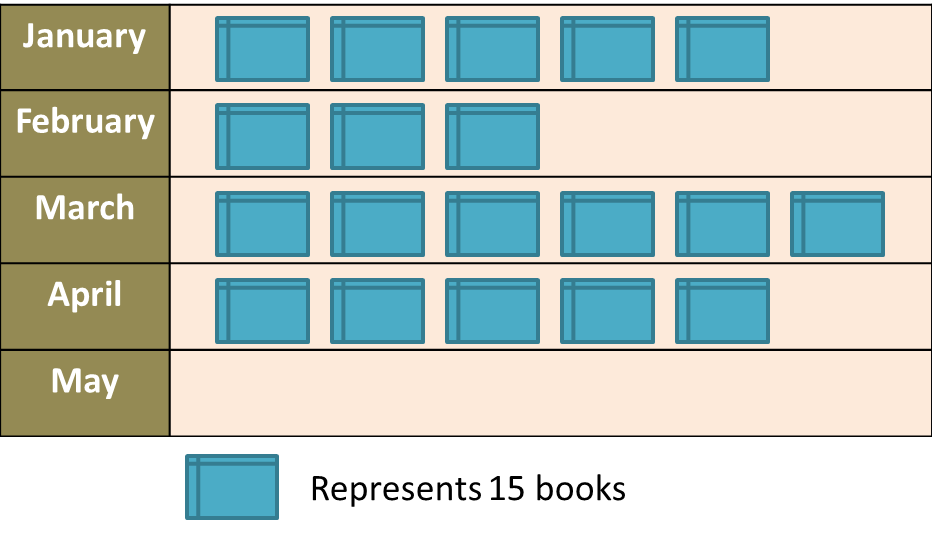(a) The sales in May is ¼ of the total sales in January and February.
Complete the pictograph in the Diagram.
(b) Find the total number of books sold before April.

Solution:
(a)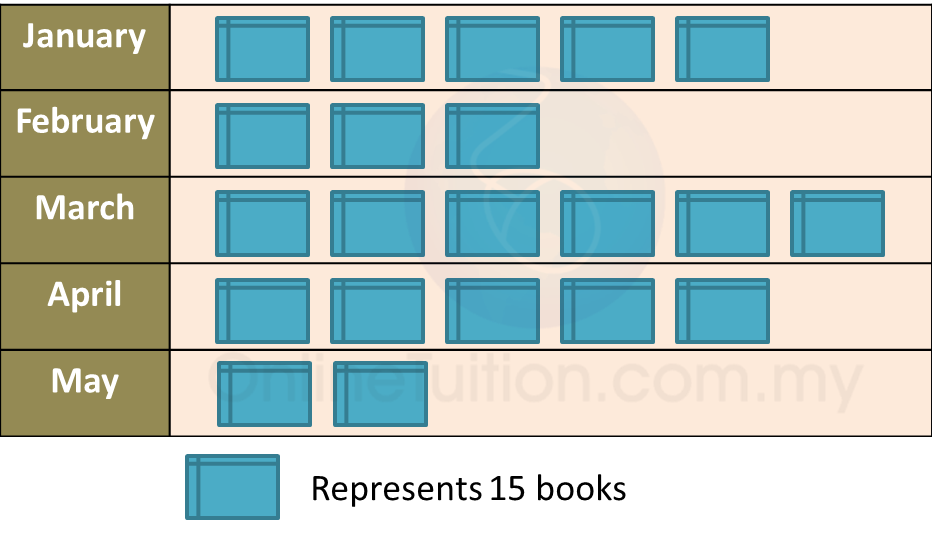(b)
Total number of books sold before April
= (5 + 3 + 6) × 15
= 14 × 15
= 210

Question 8:
Diagram below shows an incomplete line graph of the number of eggs sold in four weeks. The number of eggs sold on week 1 is 2000 and 4000 on week 4.(a) Complete the line graph in the Diagram.
(b) Complete the pie chart in the second Diagram to represent sales from Week 1 to Week 4.Solution:
(a)(b)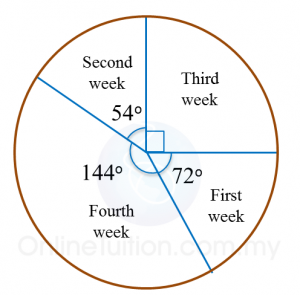# 13.2.2 Statistics (I), PT3 Focus Practice

 
 
13.2.2 Statistics (I), PT3 Focus Practice
 

 Question 4:
 
Table below shows the profit made from the sales of coconut at a stall over five days.
 

 
   Day     Day 1     Day 2     Day 3     Day 4     Day 5     Profit (RM)     280     200     200     360     320  
 
On diagram in the answer space, draw a line graph to represent all the information in the Table. Use the scale 2 cm to RM80 on the vertical axis.
 
 
: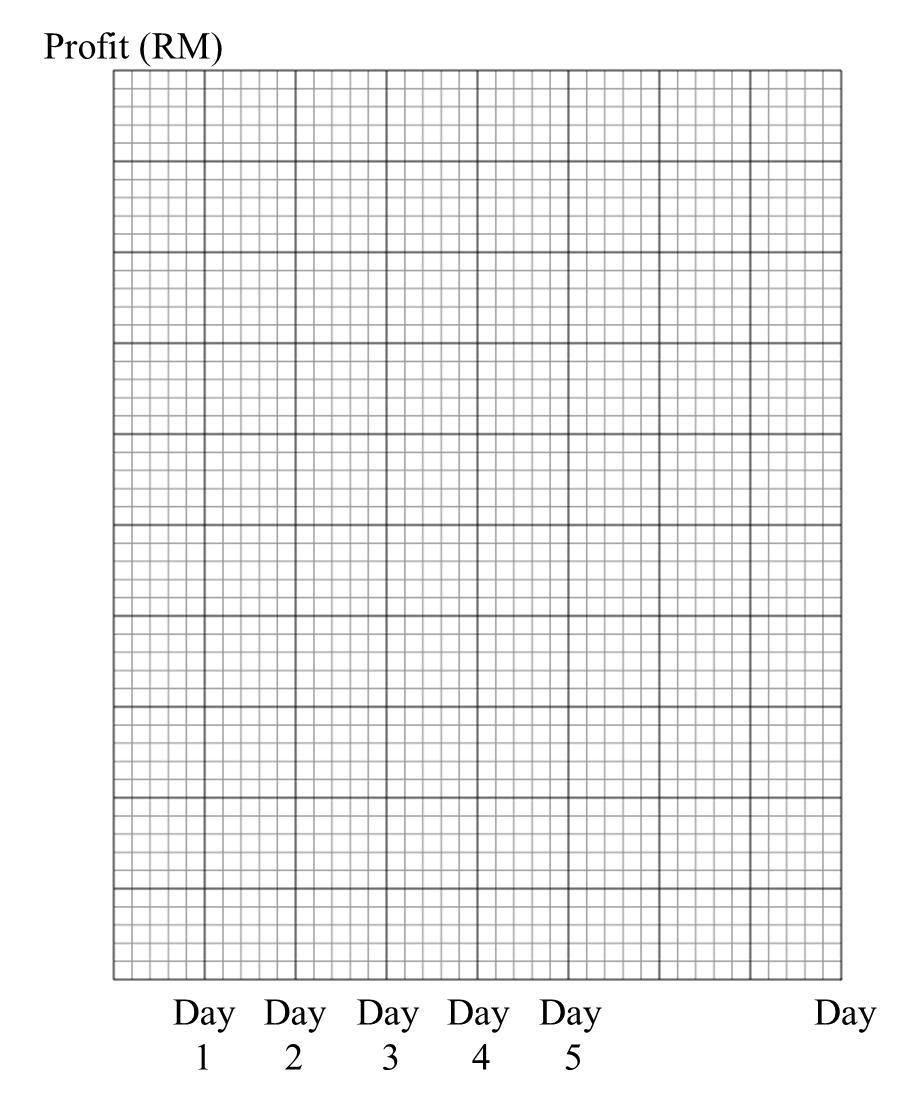Solution:Question 5:
 
Table below shows the number of students who read four types of books.
 
 
 
 
   Types of books     Fiction     Language     Technology     Religion     Number of students     18     16     8     14  
 
 
On diagram in the answer space, draw a bar chart to represent all the information in the Table.
 
 
: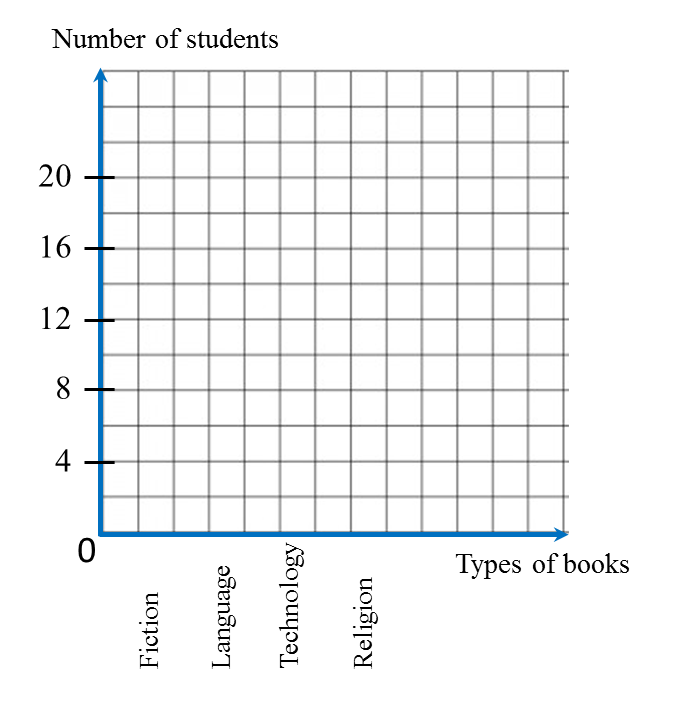Solution: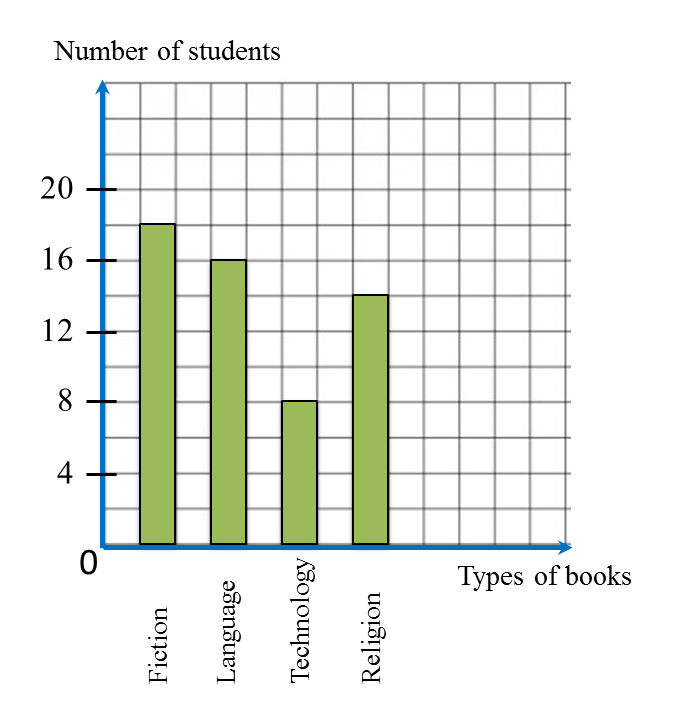Question 6:
Diagram below is an incomplete bar chart which shows the number of cars sold in a shop for duration of five months.(a) The number of cars sold for the five months is 150 units. The number of cars sold in February is equal to the number of cars sold in April.
Complete the bar chart in the Diagram.
(b) Find the percentage of cars sold in January.

Solution:
(a)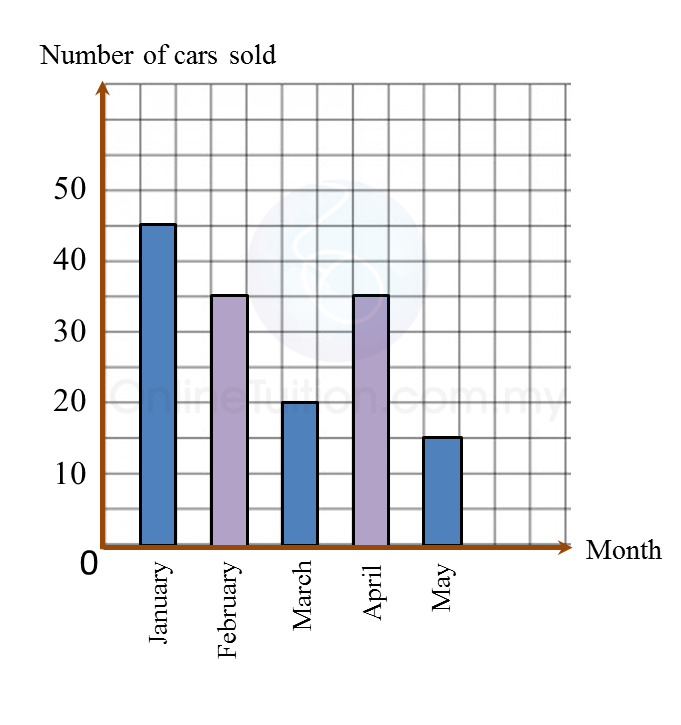(b)

# 4.7.6 Natural Polymers (Structured Questions)

Question 1:
Diagram 1.1 shows an experiment to study the effect of vinegar and ammonium solution on latex.
Diagram 1.2 shows the result of the experiment after 30 minutes.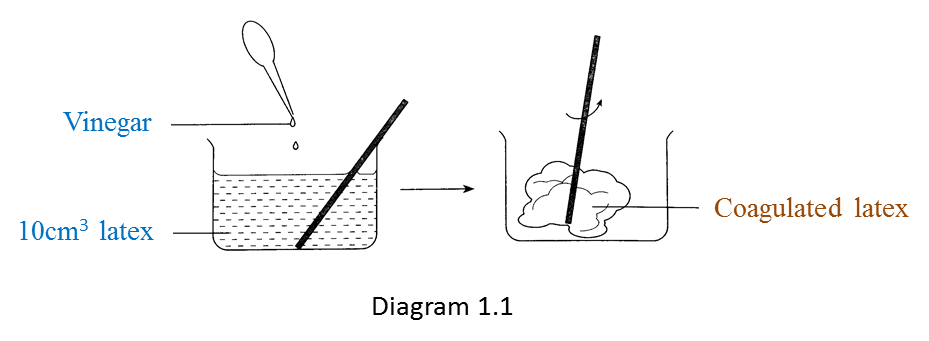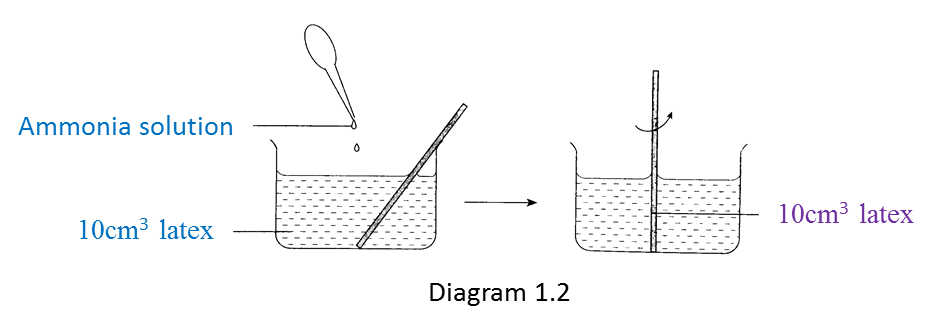(a) Write down one observation from the result of this experiment. [1 mark]

(b) State one inference based on the observation in Diagram 1.1. [1 mark]

(c) State one constant variable in this experiment. [1 mark]

(d) State one hypothesis for this experiment. [1 mark]

(e) Based on this experiment, state the operational definition for acid. [1 mark]

:
(a)
Vinegar causes the coagulation of latex but ammonia solution prevents the coagulation of latex.

(b)
When vinegar (an acid) is mixed with latex, the latex coagulates.
When ammonia solution (an alkali) is mixed with latex, the latex does not coagulate.
(any one)

(c)

1. 10 cm3 of latex
2. Results of the experiment are observed after 30 minutes
(any one)

(d)
Acid causes the coagulation of latex but alkali prevents the coagulation of latex.

(e)
Acid is a substance which causes latex to coagulate.

# 13.2.1 Statistics (I), PT3 Focus Practice

13.2.1 Statistics (I), PT3 Focus Practice
Question 1:
Diagram below is a pictograph showing the number of pizzas sold in three months.

The price of one pizza is RM18. The total sales for those four months are RM2250.
Calculate the number of pizzas sold in April.

Solution
:
Total number of pizzas sold in four months
$\begin{array}{l}=\frac{RM2250}{RM18}\\ =125\end{array}$

Total number of pizzas sold in first three months
= 9 × 10
= 90

Number of pizzas sold in April
= 125 – 90
= 35

Question 2:
Diagram below is a bar chart showing the number of new houses sold over four days.

The total number of new houses sold on Wednesday and Thursday is 56.
Calculate the total number of new houses sold on Monday and Tuesday.

Solution
:
Wednesday: 12 units
Thursday: 16 units
Number of houses represented by 1 unit
$\begin{array}{l}=\frac{56}{12+16}\\ =2\end{array}$
Monday: 14 units
Tuesday: 18 units

Total number of new houses sold on Monday and Tuesday
= (14 + 8) × 2
= 64

Question 3:
Table below shows the number of companies that gave contributions to an orphan fund over a period of five days.

 Days Monday Tuesday Wednesday Thursday Friday Number of companies 8 14 m 17 11

It is given that 20% of the total contribution was made on Tuesday.
Calculate the value of m

Solution
:
Let C = Total contribution
$\begin{array}{l}\frac{20}{100}×C=14\\ \text{}C=14×\frac{100}{20}\\ \text{}=70\\ m=70-\left(8+14+17+11\right)\\ \text{}=70-50\\ \text{}=20\end{array}$